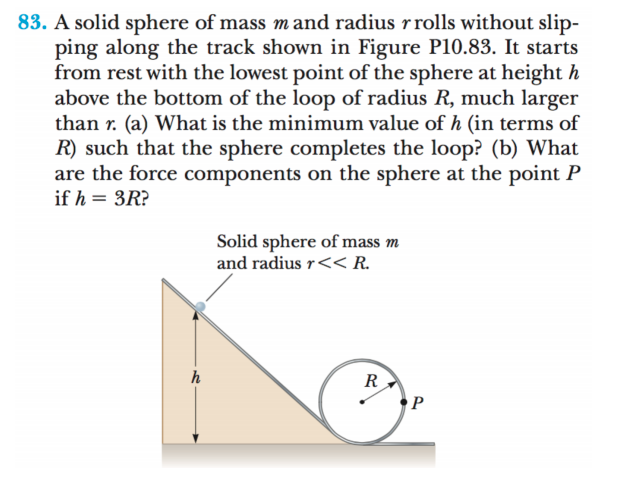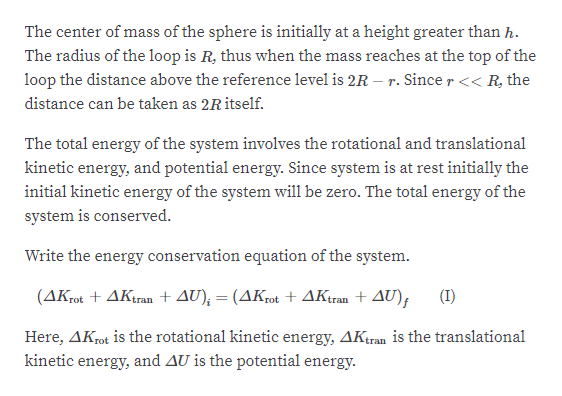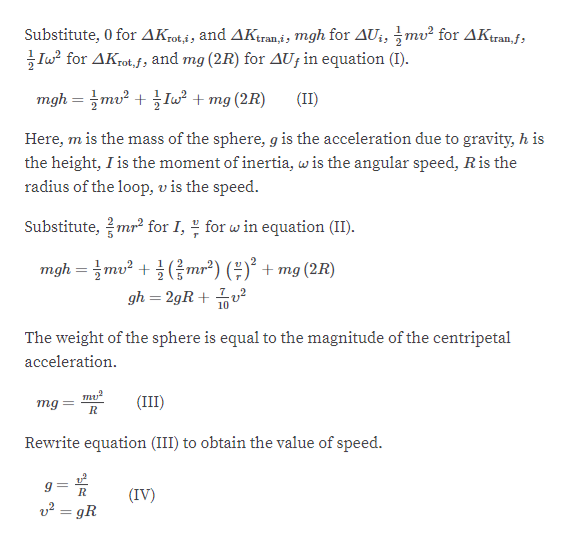# 83. A solid sphere of mass mand radius rrolls without slip-ping along the track shown in Figure P10.83. It startsfrom rest with the lowest point of the sphere at height habove the bottom of the loop of radius R, much largerthan r. (a) What is the minimum value of h (in terms ofR) such that the sphere completes the loop? (b) Whatare the force components on the sphere at the point Pif h = 3R?Solid sphere of mass mand radius r<< R.R

Question
1 viewshelp_outlineImage Transcriptionclose83. A solid sphere of mass mand radius rrolls without slip- ping along the track shown in Figure P10.83. It starts from rest with the lowest point of the sphere at height h above the bottom of the loop of radius R, much larger than r. (a) What is the minimum value of h (in terms of R) such that the sphere completes the loop? (b) What are the force components on the sphere at the point P if h = 3R? Solid sphere of mass m and radius r<< R. R fullscreen
check_circle

Step 1

Consider the figure below:

Step 2help_outlineImage TranscriptioncloseThe center of mass of the sphere is initially at a height greater than h. The radius of the loop is R, thus when the mass reaches at the top of the loop the distance above the reference level is 2R – r. Sincer<< R, the distance can be taken as 2R itself. The total energy of the system involves the rotational and translational kinetic energy, and potential energy. Since system is at rest initially the initial kinetic energy of the system will be zero. The total energy of the system is conserved. Write the energy conservation equation of the system. (ΔΚ + ΔΚ.. + Δυ), - (ΔΚ + ΔΚ+ ΔU); (I) rot Here, AKrot is the rotational kinetic energy, AKtran is the translational kinetic energy, and AU is the potential energy. fullscreen
Step 3help_outlineImage TranscriptioncloseSubstitute, 0 for AKrot,i, and AKtran,i; mgh for AU¡, mv² for AKtran,f; Iw for AKrot,f; and mg (2R) for AU; in equation (I). mgh = mv² + Iw² + mg (2R) (II) Here, m is the mass of the sphere, g is the acceleration due to gravity, h is the height, I is the moment of inertia, wis the angular speed, Ris the radius of the loop, v is the speed. Substitute, mr for I, for w in equation (II). mgh = mv² + } mr²) (÷)° + mg (2R) gh = 2gR + u² 10 The weight of the sphere is equal to the magnitude of the centripetal acceleration. mu (III) mg Rewrite equation (III) to obtain the value of speed. (IV) v2 = gR fullscreen

### Want to see the full answer?

See Solution

#### Want to see this answer and more?

Solutions are written by subject experts who are available 24/7. Questions are typically answered within 1 hour.*

See Solution
*Response times may vary by subject and question.
Tagged in

### Rotational Mechanics